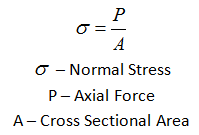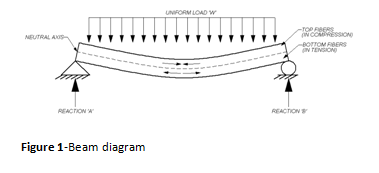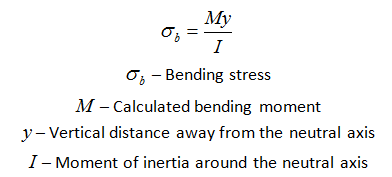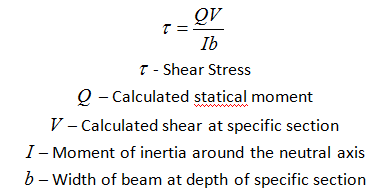# Formulae for Bending Stress, Shear Stress, and Normal Stress in Beam Calculations

## Stresses in Beams

In a separate article entitled “Key Factors for Structural Beam Calculation & Analysis,” there was a brief discussion of stresses and their function in structural analysis. In this article, we will review normal, bending, and shear stress in more depth.

## Formula for Normal Stress

A normal stress is a stress that occurs when a member is loaded by an axial force. The value of the normal force for any prismatic section is simply the force divided by the cross sectional area.A normal stress will occur when a member is placed in tension or compression. Examples of members experiencing pure normal forces would include columns, collar ties, etc.

## Formula for Bending StressWhen a member is being loaded similar to that in figure one bending stress (or flexure stress) will result.  Bending stress is a more specific type of normal stress.  When a beam experiences load like that shown in figure one the top fibers of the beam undergo a normal compressive stress. The stress at the horizontal plane of the neutral is zero.  The bottom fibers of the beam undergo a normal tensile stress.  It can be concluded therefore that the value of the bending stress will vary linearly with distance from the neutral axis.Calculating the maximum bending stress is crucial for determining the adequacy of beams, rafters, joists, etc.

## Formula for Shear Stress

Normal stress is a result of load applied perpendicular to a member.  Shear stress however results when a load is applied parallel to an area. Looking again at figure one, it can be seen that both bending and shear stresses will develop. Like in bending stress, shear stress will vary across the cross sectional area.Calculating the maximum shear stress is also crucial for determining the adequacy of beams, rafters, joists, etc.

## Beam Calculation Software Makes Stress Calculations Easier

When doing any kind of beam design using structural design software will greatly ease the entire process of calculating stresses. There are several different engineering design software packages available for beams, columns, or foundation design. If you’re in the market, try out Vitruvius with a free 30-day trial, no strings attached!